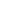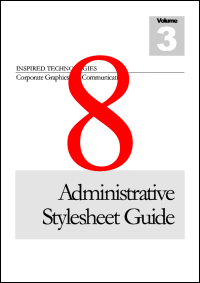Deletion ExampleThis example shows how to delete pages from a PDF document.Setup

 First, we create an ABCpdf Doc object and read our source document. We store the number of pages we're going to delete - we're going to delete all but one. Set theDoc = Server.CreateObject("ABCpdf12.Doc") theDoc.Read "c:\mypics\sample.pdf" theCount = theDoc.PageCount - 1Delete

 We go around a loop deleting the second page each time. For i = 1 to theCount   theDoc.PageNumber = 2   theDoc.Delete theDoc.Page NextSave

 We add some text to the PDF so that we know how many pages we've deleted. Finally, we save the PDF. theDoc.FontSize = 500 theDoc.Color = "255 0 0" theDoc.HPos = 0.5 theDoc.VPos = 0.3 theDoc.AddText theCount theDoc.Save "c:\mypdfs\deletion.pdf"Resultsdeletion.pdf